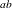### Syntax: EFFECTPLOT Statement

`EFFECTPLOT` <plot-type <(plot-definition-options)>> </ options> ;

The available plot-types and their plot-definition-options are described in Table 19.13. Table 19.15 lists the options that can be specified after a slash (/) for any plot-type, and Table 19.16 lists additional options that enhance specific plot-types. Full descriptions of the plot-definition-options and the other options are provided in the section Dictionary of Options.

Table 19.13: Plot-Types and Plot-Definition-Options

Plot-Type and Description

Plot-Definition-Options

BOX

Displays a box plot of continuous response data at each level of a CLASS effect, with predicted values superimposed and connected by a line. This is an alternative to the INTERACTION plot-type.

PLOTBY= variable or CLASS effect
X= CLASS variable or effect

CONTOUR

Displays a contour plot of predicted values against two continuous covariates

PLOTBY= variable or CLASS effect
X= continuous variable
Y= continuous variable

FIT

Displays a curve of predicted values versus a continuous variable

PLOTBY= variable or CLASS effect
X= continuous variable

INTERACTION

Displays a plot of predicted values (possibly with error bars) versus the levels of a CLASS effect. The predicted values are connected with lines and can be grouped by the levels of another CLASS effect.

PLOTBY= variable or CLASS effect
SLICEBY= variable or CLASS effect
X= CLASS variable or effect

MOSAIC

Displays a mosaic plot of predicted values by using up to three CLASS effects

PLOTBY= variable or CLASS effect
X= CLASS effects

SLICEFIT

Displays a curve of predicted values versus a continuous variable, grouped by the levels of a CLASS effect

PLOTBY= variable or CLASS effect
SLICEBY= variable or CLASS effect
X= continuous variable

By default, a single plot is produced based on the type of response variable and the number of continuous and classification covariates in the model, as shown in Table 19.14. If you have a polytomous response model, then the response variable is treated as the grouping classification variable in this table. If your model does not fit into Table 19.14, then a default plot is not produced; however, specifying the plot-type argument displays a plot with the extra continuous covariates fixed at their mean values and the extra classification covariates fixed at their reference levels.

Table 19.14: Default Plot-Types

Number of Covariates

Type of Response Variable

Classification

Continuous

Continuous or Binary

Polytomous

1

0

2

0

None

0

1

0

2

None

1

1

None

Table 19.15 and Table 19.16 list the options that can be specified after a slash (/) to enhance the effect plots.

Table 19.15: Available Options for All Plot-Types

 NROWS=INDIVIDUALNCOLS=LINKNOOBSNot available for the BOX plot-type.Not available for the MOSAIC plot-type.

Note: If your model contains an offset variable and the MOFF option is not specified or not valid, then the predicted values are computed only at the observations. In this case, the FIT and SLICEFIT plot-types display scatter plots of the predicted values, the CONTOUR plot-type displays the residuals against two continuous covariates but with no fitted surface, the INTERACTION plot-type does not connect the predicted values with lines, and the BOX plot-type is unchanged.

Table 19.16: Additional Options for Each Plot-Type

 BOX CONTOUR Plot-Type Options